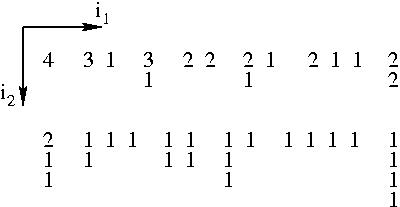## Partitions of an Integer

 Linear partitions A 1-dimensional or linear partition of an integer is a decomposition into a sum of positive integers in which the summands are ordered from largest to smallest. For instance, the linear partitions of 5 are 5 = 4 + 1 = 3 + 2 = 2 + 2 + 1 = 2 + 1 + 1 + 1 = 1 + 1 + 1 + 1 + 1. Plane partitions A 2-dimensional or plane partition of an integer is a decomposition into a sum of smaller positive integers which are arranged on a plane. The ordering property generalises to the summands being non-increasing along both the rows and the columns. The plane partitions of 4 are shown below.Higher dimensional partitions. Now, the summands are arranged on a d-dimensional hyper-cubic lattice. The ordering property generalises to the summands being non-increasing along all the lattice directions. The 3-dimensional partitions are also known as solid partitions An enumeration problem. How many ways can one partition an integer? The answer (for the generating function) is known for linear partitions and plane partitions. But nothing much is known about higher dimensions. What I have been looking at. Using exact enumeration and monte carlo methods, Ville Mustonen and I calculated numerically the asymptotic behaviour of solid partitions of an integer. We showed that an old conjecture of P.A.MacMahon could give the right asymptotic behaviour (paper). We also enumerated all solid partitions for integers up to 50 (list). Currently I am working on a simple combinatorial proof for the MacMahon formula for plane partitions. References Two references that are easily accessible to physicists: 1. G .E. Andrews 1976 The Theory of Partitions, Encyclopedia of Mathematics and its Applications Vol 2 (Addison-Wesley, Reading) 2. R. P. Stanley 1999 Enumerative Combinatorics, Vol. 2 (Cambridge University Press, Cambridge)